## Introduction

Turbomachinery blades are susceptible to non-uniform flows generated by inlet distortions, wakes and upstream and downstream pressure disturbances from adjacent blade rows. Pressure non-uniformity is particularly strong in the fan bypass duct due to the existence of pylons and struts which support the fan casing. The potential field disturbances of these obstacles in the form of a circumferentially varying pressure can result in forced excitation of the fan rotor, noise generation and increased aerodynamic losses. These pressure waves can eventually propagate upstream through the fan, and as a result, rotor blades are subject to unsteady fluctuations causing the fan to operate with a non-uniform exit pressure. Operation in a distorted flow-field also affects fan performances.

Analytical models were used for the rotor and stator rows by some researchers (Kodama, 1985) used a two-dimensional compressible semi-actuator disk model based on the theory of small perturbations for a fan stage. Static pressure disturbances due to downstream pylons or struts were separately obtained and imposed at the exit of the fan stage model. However, time-accurate CFD simulations are frequently used in design-optimization systems and are becoming more and more widespread within the industry (Rife et al., 2018; Di Mare et al., 2019). Since the mechanism of rotor interaction with a downstream potential pressure field is inherently unsteady, URANS analyses of a 360° full computational model of the whole stage with downstream obstructions is required to correctly resolve the problem (Enoki et al., 2013) performed three-dimensional unsteady viscous analyses for two fan rotor-stator-pylon configurations with different axial gaps between the stator and the pylon, and compared their results with experimental data. The common characteristic of these numerical strategies based on URANS simulations is their high computational cost. Modeling the aerodynamic effects using full-annulus transient simulations though feasible (Shahpar et al., 2003; Oishi et al., 2012), is still too expensive for routinely used in design offices.

Turbomachinery flows are characterized by their spatial cyclic symmetry and numerical methods taking advantage of it have been proposed to reduce their complexity and cost. Many problems of interest are characterized by two distinctive separate scales and a large multiplicity of similar small-scale elements. In a block-spectral approach, the global domain is decomposed into a large number of similar small blocks making use of scale-dependent solvability. The use of spatial Fourier methods for the computation of nonlinear flows in turbomachinery was first proposed by He (2006). In his original work, He used a block decomposition to study the steady 2D interaction between a fan OGV and a Pylon. Moreover, simplified unsteady cases with two-dimensional (He, 2006) or axisymmetric rotor disk cavities (He, 2011b) were expanded in spatial Fourier series and subject to long-wavelength unsteady perturbations showing good agreement between the spectral method and the full annulus solutions with significant computational savings. He applied the same idea to film cooling configurations (He, 2011a), dimples (He, 2013a) and lined acoustic intakes (He, 2013b). Recently (He, 2018), also extended the methodology to unsteady flows of short temporal and spatial scales (e.g. those due to self-excited unsteady vortices and turbulence disturbances), where a source term-based approach was adopted to facilitate a two-way coupling in terms of time-averaged flow solutions. The present work exploits the same idea extending and applying the spatial Fourier modeling to 3D non-axisymmetric unsteady airfoil passages under the presence of long-wavelength flow instabilities without making any explicit assumption about the temporal periodicity of the flow.

A time-marching Passage-Spectral Method (PSM) is proposed in this study to reduce the computational cost of these unsteady and non-axisymmetric simulations and simplify the study of the fan rotor stability under the effect of a distorted exit static pressure without making any hypothesis about its temporal periodicity. The idea is essentially the same presented by He (2006). The Passage-Spectral naming is solely used a means of highlighting that the blocks coincide with the blade passages. This approach, which has been verified against full-annulus cases for rotating stall and force response cases in an isolated fan-stage (Romera and Corral, 2020a), has been further modified to deal efficiently with a single spatial harmonic and then applied to assess the effect of inlet distortion on fan stability (Romera and Corral, 2020b). Despite the number of struts or pylons and their geometrical complexity, any outlet static pressure distortion pattern can be Fourier decomposed. The stability of the spatial harmonics content can the be assessed separately using the proposed PSM filtering the contribution of the rest of the circumferential modes of the downstream perturbation. The validity of this approach has been shown by (Romera and Corral, 2020b). In this model, just three reduced-passages are retained. The proposed method assumes that the circumferential wavelength of the potential disturbances is much larger than the blade spacing. In this paper first, the nonlinear stability of each nodal diameter at the outlet is investigated as a function of the perturbation amplitude taking advantage of the use of the novel method. Next, full-annulus simulations are carried out to increase the credibility of the stability maps. In the authors’ opinion, this is the first time clear conclusions are drawn about the influence of the outlet distortion shape on fan stability.

## Numerical setup

The baseline solver, known as Mu2s2T (Burgos et al., 2011), uses hybrid unstructured grids to discretize the spatial domain and can contain cells with an arbitrary number of faces. The three-dimensional Navier-Stokes equations in conservative form for an arbitrary control volume are discretized using a finite volume approach based on the dual mesh where the solution vector stores the variables at the vertexes of the mesh. The control volume associated with a node is formed by connecting the median dual of the cells surrounding it, using an edge-based data structure. The spatial discretization is formally second-order accurate and a second-order backward implicit time integration is used. A matricial form of the numerical dissipation terms is used recurring to the Roe’s matrix. The code is parallelized using MPI and executed in GPUs to reduce the turn around time (Corral et al., 2017).

The existing parallel CFD code was modified and customized for this work to study the contribution of a given single spatial harmonic M efficiently, by approximating the solution with only three reduced-passages samples spanning over a sector of size 2π/M. Reader is referred to reference Romera and Corral (2020a) and Romera and Corral (2020b) for further details of the baseline flow solver and the implementation of the proposed method.

The code is based on the virtual existence of a Fourier transformation of the homologous nodes of the different passages, Uij, and the description of the flow in terms its Fourier coefficients:

##### (1)
U^im(x,t)=1NJj=1NJUi(j)(x,r,θj,t)eImθj

where i represents homologous nodes in different j-th uniformly spaced passage

##### (2)
θj=j1MNJ2π

being NJ the number of passages retained in the Fourier transform, which in this work is NJ=3, since only three reduced-passages are needed to approximate the first spatial harmonic inside each 2π/M sector size. The purpose of using a Fourier modeling of the spatial flow-field is to use a truncated Fourier series of the “exact” solution and retain only the most representative harmonics. The underlying hypothesis is that the wavelength of the circumferential non-uniformities is long, and therefore, a few harmonics suffice to represent the long wave-lengths. Short wave-length variations are retained within the blade passage mesh. If only a single arbitrary harmonic of the perturbed flow is retained then just three equally spaced passages need to be computed to reconstruct the mean value and the harmonic. Once the solution of the uniformly spaced three reduced-passages are calculated at each time step (see Figure 1), the full-annulus solution can be obtained decomposing the flow variables at all nodes at the inner domain solution of the sample passages into spatial Fourier components and reconstructed on the full circumference with the corresponding phase-shift, which is equal to the pitch of the reduced-passage sample.

#### Schematic of a sinusoidal distribution in full-annulus (left) and its approximation (right) with three reduced-passages samples (dark grey) uniformly spaced.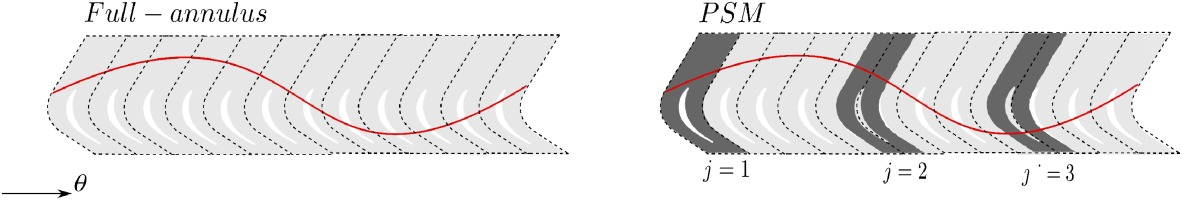Once the solution of the three uniformly spaced reduced-passages is calculated at each time step (see Figure 1), the reconstructed full-annulus solution can be obtained Fourier decomposing the flow variables at all the nodes of the sample passages. Once the Fourier coefficients at all the nodes of the passage are obtained these can be used to expand the solution to the full circumference. Since the reduced-passage samples play the role of sampling points for the circumferential Fourier transform and require uniform spacing, the circumferential location of these sample passages does not correspond in general with the position in the full-annulus of the physical blades or passages. The Fourier coefficients use as reference the first reduced-passage (j=1 in Figure 1), which has the same physical location as the original full-annulus passage. The solution at the rest of the blades/passages of the full circumference is reconstructed using the Fourier coefficients. This step is only needed for displaying purposed or post-processing.

## Fan stability

This section aims at studying the nonlinear aerodynamic stability of a generic fan blade operating at a constant speed under the potential effect caused by a circumferential distribution of static pressure at the outlet, which is parameterized in terms of its nodal diameter and static pressure amplitude. The performances and stability of axial flow fans and compressors is affected by the circumferential variations in exit static pressure produced by thick struts or other components if they are not located far enough from the fan rotor. These flow disturbances are usually dominated by long circumferential wave-lengths (i.e. much longer than the blade pitch) which makes the Passage-Spectral Method well suited for their analysis since it avoids the use of full-annulus simulations. Moreover, the method allows investigating the independent contribution of the different NDs efficiently. It is important to point out that this is not the case in nonlinear full-annulus simulations since when the perturbations are large, and the linear stability regime is by-passed, the nonlinear interaction amongst the different NDs is unavoidable.

In this regard, the method has been adapted to simulate an arbitrary single ND perturbation, simulating the equivalent effect of different number of pylons downstream, using solely three reduced passages to fit the desired wave-length over a sector of 2π/ND degrees, filtering the nonlinear contribution of the rest. Two types of simulations are used in this work. The first are full-annulus simulations which are used as a high-order model for verification purposes. The second are PSM simulations with a single spatial harmonic or ND matching that of the outlet static pressure perturbation. This approach is very efficient and is used to map the design space.

### Numerical set-up

The NASA rotor 67, which is a low-aspect-ratio fan with 22 rotor blades, has been chosen as the test vehicle. Figure 2 summarizes the fan design parameters at 100% of the design speed. The rotor blade is discretized using a semi-structured grid (Burgos et al., 2011). Firstly, a hybrid grid in the blade-to-blade plane with 118 points around the airfoil and 24 quad layers in the boundary layer has been constructed. Next 151 layers in the span-wise direction are extruded. To resolve the tip leakage flow 6 mesh layers were included between the blade tip and the casing. The final grid consists of about 3.53×106 nodes per passage. The size of the full-annulus mesh is about 78×106 nodes. A duct of approximately 0.3D and 0.3D has been added upstream and downstream of the rotor respectively to ease fan stability analysis although it is acknowledged that the stability margin depends on this location (Zhang and Vahdati, 2018). It was concluded that as the exit duct becomes longer, the stall margin loss of the blade increases.

### NASA rotor 67 speed-line from PSM simulations and design parameters.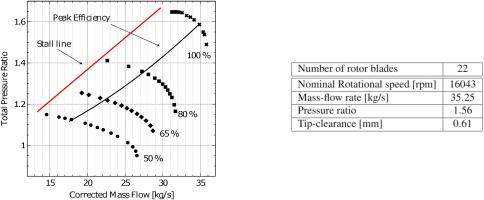One-dimensional non-reflecting boundary conditions were imposed at the inlet and the outlet. At the exit, the radial equilibrium equation was applied to fix the pressure radial distribution. Non-slip and adiabatic wall boundary conditions were applied to the blade surfaces and the inner and outer casing walls. The Reynolds number of the simulations is very high (Re106) and the standard kω turbulence model with wall functions was used to alleviate mesh requirements in the boundary layers. Figure 3 displays the computational domain and the three reduced-passages required to carry out the spatial Fourier transformation for a single spatial harmonic and the whole length of the computational domain. Figure 2 represents the different operating conditions in the fan map. The steady solution at 100% of the design speed with uniform static pressure in the radial direction at the outlet at a near peak efficiency operating point is used as the initial solution for the unsteady simulations.

### NASA rotor 67 full computational domain (3 reduced-passages) for a ND=1 approximation.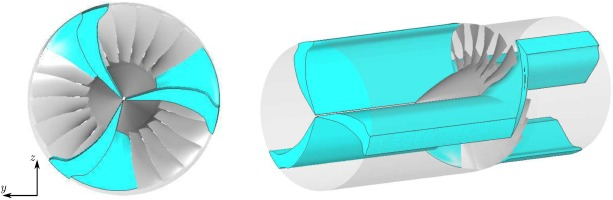The mass flow evolution for three different time resolution (200, 400 and 4,000 time steps/rev) has been analyzed. We concluded that 400 time steps per rotor revolution is sufficient to predict the stall margin. It should also worth mentioning that the stall margin is greatly influenced by the size of the time step (Zhang and Vahdati, 2017). The use of a large time steps over-predicts the stall margin, delaying the stall inception at a given mass flow of reference. Just to conclude, unsteady simulations have been conducted using 400 time steps per rotor revolution. A previous analysis was also done for the number of pseudo time steps per physical time step, fixed to 60, which is large enough to achieve the convergence criterion of the inner iteration of the dual time step. The base flow is uniform and axial at the outlet, but the static pressure is modified circumferentially by superimposing an arbitrary Fourier series but keeping the radial distribution of the static pressure.

### Stability map

This section aims at studying the influence of downstream circumferential static pressure patterns on fan stability to shed some light on the influence of the pylon shape and number off in the non-linear stability of a fan-stage. A computationally efficient method is used to enable this analysis during the conceptual design phase of the fan. The nonlinear stability of the fan is then investigated in a nodal diameter basis retaining the spatial harmonic corresponding to the outlet static pressure perturbation solely. The pressure ratio is modified by changing the mean level of the circumferentially average exit static pressure.

The effect of circumferentially varying the amplitude of the outlet static pressure for the first four nodal diameter patterns on the fan stability is investigated with the Passage-Spectral Method at Ωrotor/Ωnominal=100%. In this case, the static pressure at the outlet can be expressed as ps(r,θ)=p¯s(r)+Δpssin (Mθ), being M the harmonic index retained in the method. The static pressure distribution at the outlet is non-uniform in the radial direction as a consequence of the swirl. However, the circumferential perturbation is superimposed to this uniform static pressure distribution in the radial direction, p¯s(r). We only modify the circumferential distribution, remaining the radial distribution invariant. Solutions are obtained using the PSM just retaining the harmonic M=ND, as shown for instance in Figure 3 for ND=1, where the three reduced-passages used in the PSM simulation are highlighted in blue. It is noteworthy that these perturbations have a traveling-wave form in the rotor frame of reference, and for the frequencies and nodal diameters studied in this work. These waves are cut-on and develop upstream without attenuation, at least for the frequencies and nodal diameters studied in this work.

The study of the amplitude effect of a static pressure perturbation downstream on the fan has been carried out taking into account realistic circumferential patterns previously reported in the literature. (Enoki et al., 2013) conducted aerodynamic and acoustic tests for a transonic fan stage with a simulated top pylon. The potential pressure field that was created by the engine pylon was about 15% of the mean static pressure three chords downstream from the fan. Taking into account the actual static pressure at the outlet of the simulations carried out at the aerodynamic design point, the amplitude of the static pressure perturbation at the exit has been varied between Δps=0 and 20.000Pa for different nodal diameters.

The nonlinear stability maps for the first four NDs computed with the PSM are shown in Figure 4. The unstable regions are shaded in color. The blue circle with the minimum mass-flow coincides with the last converged point with the single-passage steady version of the solver. At this OP the fan is unstable under infinitely small perturbations. As the mass-flow is increased the fan is more tolerant of outlet perturbations. The stability of the first and third NDs, see Figure 4a and c, are practically not altered by the perturbation level. These cases exhibit nearly straight vertical lines departing from the same point, at least within the error of the method, which is the linear or small perturbation stability limit. This instability process is nonlinear, since the perturbations have finite amplitude, and for small perturbations, this phenomenon cannot be seen. Its simulation requires of fully nonlinear analyses.

### Stability conceptual map due to different amplitudes of static pressure patterns at the outlet and nodal diameters using the PSM just retaining the harmonic M=ND at 100% of the design speed. (a) ND=1, (b) ND=2, (c) ND=3 and (d) ND=4.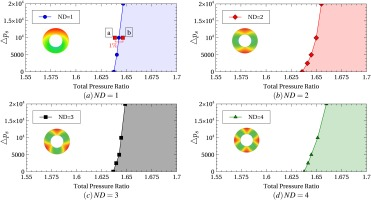It can be seen that the contribution of the different NDs to nonlinear stability is qualitatively different. The most surprising result of Figure 4 is the stabilizing behavior of ND=2 and 4. The stability boundary of these nodal diameters exhibits a supercritical bifurcation, i.e. OPs which are unstable under small perturbations at the outlet, Δps/ps1, can become stable in the presence of large disturbances at the outlet. The actual interpretation of Figure 4b and d is that the fan could operate beyond the linear stability limit if the sole potential effect at the outlet would correspond to the ND=2 or 4, and the amplitude of the static pressure disturbance at the exit is high enough. In this case, the fan exhibits a periodic but stable response to this type of potential effect downstream on the fan. The combination of these stable NDs with other NDs, such as the first, has not been further pursued. Comparing the results for the first four NDs in Figure 4 can be concluded that the first and the third NDs have the largest sensitivity to outlet static pressure perturbations, and in practice, their behavior is the one observed with realistic, non-sinusoidal, perturbations.

The stability of the fan-stage under small perturbations is studied next. The simulations are set with a constant circumferentially uniform static pressure at the exit (Δps=0 in Figure 4) and a single harmonic, M, of the PSM. This is equivalent to compute different sector sizes, as shown in Figure 3 for the M=1, or in the insets of Figure 4. It can be seen that the most unstable ND is the third, but the difference between different NDs is very small. It is concluded that the critical pressure ratio derived from the small perturbation analysis has a weak dependence with the ND and the departing point of all the nonlinear stability limit for all the NDs is essentially the same.

This type of plot is difficult to construct using full-annulus simulations, especially if the stability limit of the different modes is similar in the parameter space since it is difficult to control and track the development of the different unstable NDs when they are active simultaneously in a single simulation. However, Figure 4 suggests that if high enough perturbations with ND=2, and 4 are introduced the fan could operate in a stable manner beyond this point, which is an interesting conclusion of this study due to its potential application. Finally, it is important to highlight that, the most critical ND is the first and the one which can be triggered by a finite-amplitude perturbation in the linearly stable region.

It is illustrative to compare these results with the more classical problem of intake distortion where a total pressure defect is set at the inlet. This problem has been addressed as well by (Romera and Corral, 2020b) using the NASA rotor 67 fan as well and following the same approach. In that case, the ND=1 total pressure perturbation at the inlet has a sub-critical bifurcation while here the sensitivity of the fan to the amplitude of a potential perturbation at the exist is very small. The most surprising result for the case of intake distortion is the strongly stabilizing behavior of a ND=3 total pressure distortion pattern at the inlet. This stabilizing effect can be shown also here for a potential perturbations at the exit with ND=2 and 4 patterns, see Figure 4, but this effect is much stronger in the case of intake distortion. It can be concluded that the fan stability sensitivity to finite amplitude periodic perturbation is much higher for intake distortion case than for potential perturbations associated to strut or pylons at the exit.

### Validation and results

Full-annulus simulations have been conducted aiming not only at validating the results obtained in the previous section but also to show the transient behavior when the flow-field becomes unstable. Steady solutions at 100% of the design speed with uniform outlet static pressure are used as the initial solution for the unsteady simulations. The full-annulus solutions obtained for the points marked as a and b in Figure 4a are discussed next. These two OPs have a slightly different pressure ratio, PR=1.64 and PR=1.65, and a 1st ND potential perturbation at the exit with an amplitude of Δps=10.000Pa. First, the stability threshold derived using PSM simulations has been validated using full-annulus simulations at these operating conditions. The point a is stable while the point b is not. This is in good agreement with the predictions of the PSM. The bracket of the error in terms of total pressure with respect the full-annulus simulation is below 1%, as shown in Figure 4a.

Figure 5a compares the mass-flow time evolution during the first 20 revolutions at the rotor inlet for the operating points a and b, corresponding to pressure ratios of PR=1.64 and PR=1.65, respectively. For the stable case (operating point a), it can be seen how following the initial perturbation the mass-flow converges to a stable periodic solution, though the simulation is fully unsteady, whereas for the unstable case (operating point b) the mass-flow drops by about 50% after approximately 12 rotor revolutions and has a chaotic behavior, see Figure 5a for the PR=1.65 case.

### Full-annulus comparison between stable and unstable conditions with a first nodal diameter (ND=1) perturbation with a static pressure amplitude at the outlet of Δps=10,000Pa, at two total pressure ratio of PR=1.64 and PR=1.65 marked as a and b in Figure 4a, respectively. Mass flow comparison (left) and pressure signal (right) at 0.5 axial chords downstream of fan near the casing for the 1st harmonic component (m=1) non-dimensionalized with the distortion amplitude at the outlet, Δps.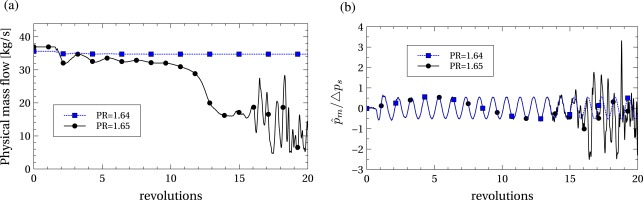Figure 5b displays the time evolution of the first circumferential harmonic (m=1) of the static pressure during the first 20 revolutions in a numerical probe located near the outer casing 0.5 axial chords downstream of the fan. The evolution of both signals in Figure 5b corresponds to the stable and unstable cases at the two different operating points, a and b, previously mentioned. As can be seen in Figure 5b the effect of imposing a static pressure circumferential distortion at the outlet is seen by the rotor as an upstream traveling-wave and sensed in the numerical probe. The stable case gives rise to a periodic disturbance in the flow as it could be expected. The amplitude of this perturbation is smaller than the imposed amplitude of the static pressure perturbation at the outlet (Δps=10.000Pa) meaning that the pressure wave traveling upstream has been attenuated. This is in agreement with the results and conclusions derived by other researchers using either numerical and experimental data (Enoki et al., 2013) or an actuator disk model (Kodama, 1985).

After approximately 15 rotor revolutions, the first harmonic of the static pressure at the numerical probe located downstream of the fan blade is triggered and experiences rapid exponential-like growth, as shown in Figure 5b, which indicates a rapid development of the non-axisymmetric flow in this region. Once the stall process is triggered, the first harmonic (m=1) is shown to be persistent in time during the next 5 rotor revolutions. Here only the time evolution of the first harmonic is shown since is the most unstable one and in consequence the most relevant for the stall breakdown of the fan-stage. At this unstable operating conditions, PR=1.65, the circumferential static pressure distortion with ND=1 and amplitude of Δps=10.000Pa triggers an unstable behavior of the fan blade that further develops into a fully stalled flow.

### Computational efficiency

The speedup factor, R, of the PSM with respect a full-annulus simulation is directly related to the ratio between the number of reduced-passages samples used to approximate the simulation, and the number of blades, which in this case is 22, since the overhead of the method is negligible. The speedup factor with respect the direct full-annulus solution using three reduced-passages samples in this case is then R=22/3=7. Table 1 compares the computational effort requires for the new methodology against a full-annulus simulation.

### Computational cost of different numerical approximations for NASA rotor 67 case under the effect of potential perturbations downstream on the fan for a mesh of 3.53×106 nodes per passage.

PSMFULL
Number of points10.6×10678×106
Number of GPUs216
Wall clock time per revolution (h)1.31.4

## Conclusions

Unsteady simulations have been carried out for the NASA rotor 67 fan blade to investigate its aerodynamic stability under the effect of potential disturbances induced by pylons and struts located downstream of the fan. The reduced-order Passage Spectral Method and full-annulus representations of the fan have been used. The PSM has been slightly modified aiming at efficiently handling outlet static pressure distortion in a nodal diameter by nodal diameter basis filtering the nonlinear contribution of the rest of the nodal diameters.

The proposed methodology is based on the heuristic assumption that the spatial harmonics of the outlet static pressure perturbations can be Fourier decomposed and evolve independently, even in the nonlinear stability regime. However, the method makes no hypothesis about the interaction between the different spatial modes. The method is fully nonlinear. In this case, independent nonlinear stability maps for each nodal diameter can be constructed. The presence of second and fourth nodal diameters has a nonlinear stabilizing effect on the fan rotor blades. The stability of the fan is not altered by the presence of finite-amplitude first and third nodal diameter perturbations. The stability threshold for one of the distorted static pressure patterns has been assessed using full-annulus simulations. The error has been estimated at about 1% in terms of total pressure ratio. This conclusion has been derived using an iterative procedure involving full-annulus solutions. If just the four first NDs are computed using the PSM this analysis can be conducted overnight in a modest cluster with 16 GTX 1080ti GPUs with a significant reduction in computational resources with respect to full-annulus analyses.

## Nomenclature

D

rotor diameter

M

number of spatial harmonics

m

spatial harmonic index

NJ

number of reduced-passages samples

ND

nodal diameter

ps

outlet static pressure

PR

total pressure ratio

R

speedup factor

U

vector of conservation variables

U^

n-th spatial harmonic of U values

x

cylindrical coordinate vector

θ

### Acronyms

CFD

Computational Fluid Dynamics

OP

Operating Point

PSM

Passage-Spectral Method

URANS